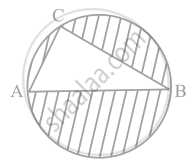# In figure, AB is a diameter of the circle, AC = 6 cm and BC = 8 cm. Find the area of the shaded region (Use π = 3.14). - Mathematics

Sum

In figure, AB is a diameter of the circle, AC = 6 cm and BC = 8 cm. Find the area of the shaded region (Use π = 3.14).#### Solution

Identify the figure as a circle, and a right-angled triangle (and semicircle, segment also), because AOB is diameter and angle in semicircle, is 90°.

So, ∠C = 90°

In right angled ΔABC,

b = base = BC = 8 cm

a = altitude = AC = 6 cmBy Pythagoras theorem in right ΔABC,

AB2 = BC2 + AC2

= 82 + 62 = 64 + 36

⇒ AB2 = 100 cm

⇒ AB = 10 cm

Hence, r = 10/2 = 5 cm

∴ Area of shaded region = Area of circle – Area of right ΔABC

= pir^2 - 1/2 Base × Alt.

= 3.14 xx 5 xx 5 - 1/2 xx 8 xx 6

= 3.14 xx 25 - 8 xx 3

= (78.50 – 24) cm2

= 54.50 cm2

∴ Area of shaded region = 54.50 cm2

Concept: Areas of Combinations of Plane Figures
Is there an error in this question or solution?

#### APPEARS IN

NCERT Mathematics Exemplar Class 10
Chapter 11 Area Related To Circles
Exercise 11.3 | Q 7 | Page 126
Share